# Difference between revisions of "Amitsur-Levitzki theorem"

A basic result in the theory of polynomial identity rings (PI-rings). A ring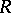is a PI-ring (cf. also PI-algebra) if there is a polynomial in the free associative algebra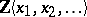which vanishes under all substitutions of ring elements for the variables. The standard polynomial of degreeis the polynomial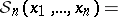where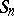is the symmetric group onletters. Since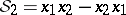, a ring is commutative if and only if it satisfies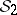(cf. also Commutative ring). The Amitsur–Levitzki theorem says that the ring of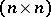-matrices over a commutative ring satisfies the standard polynomial of degree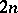, and no polynomial of lower degree.

There are five different published proofs of this theorem (1996). The original proof by S. Amitsur and J. Levitzki [a1] is a combinatorial argument using matrix units. Because the standard polynomial is linear in each variable, it is enough to prove the theorem when matrix units are substituted for the variables. A streamlined version of this proof can be found in [a3].

The second proof, by B. Kostant [a2], depends upon the Frobenius theory of representations of the alternating group. Kostant's paper was also the first to relate the polynomial identities satisfied by matrices with traces, a theme which was later developed by C. Procesi [a4] and Yu.P. Razmyslov [a5] and influenced much research. The point is that the trace defines a non-degenerate bilinear form on-matrices.

The third proof, by R.G. Swan [a7], translates the problem into graph theory. The underlying arguments are similar to those in the original proof by Amitsur and Levitzki, but the graph-theoretical approach has led to some generalizations, as in [a8]. The fourth proof, by Razmyslov [a5], is probably the most natural, in that the theorem is deduced in a direct way from the multilinear form of the Cayley–Hamilton theorem. The fifth proof, by S. Rosset [a6], also depends on the Cayley–Hamilton theorem, as well as on elementary properties of the exterior algebra. It is the shortest and most elegant proof, but is not at all straightforward.

How to Cite This Entry:
Amitsur-Levitzki theorem. Encyclopedia of Mathematics. URL: http://encyclopediaofmath.org/index.php?title=Amitsur-Levitzki_theorem&oldid=22019
This article was adapted from an original article by E. Formanek (originator), which appeared in Encyclopedia of Mathematics - ISBN 1402006098. See original article